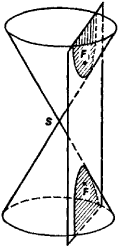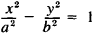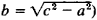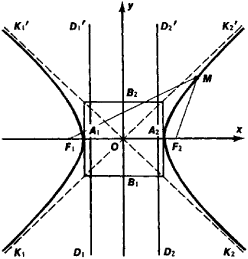# hyperbola

Also found in: Dictionary, Thesaurus, Wikipedia.

## hyperbola

(hīpûr`bələ), plane curve consisting of all points such that the difference between the distances from any point on the curve to two fixed points (foci) is the same for all points. It is the conic sectionconic section
or conic
, curve formed by the intersection of a plane and a right circular cone (conical surface). The ordinary conic sections are the circle, the ellipse, the parabola, and the hyperbola.
formed by a plane cutting both nappes of the conecone
or conical surface,
in mathematics, surface generated by a moving line (the generator) that passes through a given fixed point (the vertex) and continually intersects a given fixed curve (the directrix).
; it thus has two parts, or branches. The center of a hyperbola is the point halfway between its foci. The principal axis is the straight line through the foci. The vertices are the intersection of this axis with the curve. The transverse axis is the line segment joining the two vertices. The latus rectum is the chord through either focus perpendicular to the principal axis. The asymptotes are lines, in the same plane, which the curve approaches as it approaches infinity. An equilateral, or rectangular, hyperbola is one whose asymptotes are perpendicular. A second hyperbola may be drawn whose asymptotes are identical with those of the given hyperbola and whose principal axis is a perpendicular line through the center; the two hyperbolas thus related are called conjugate.

## hyperbola

(hÿ-per -bŏ-lă) A type of conic section that has an eccentricity greater than one. See also orbit.

## Hyperbola

The section of a right circular cone by a plane that intersects the cone on both sides of the apex.

## Hyperbola

the curve of intersection of a circular cone with a plane cutting both of its nappes (Figure 1). A hyperbola may also be defined as the geometric locus of the points M in a plane, such that the difference of their distances from two fixed points F1 and F2 (foci of the hyperbola) in that plane is constant. If a coordinate system xOy is selected such asFigure 1

that represented in Figure 2 (OF1 = OF2 = c), then the equation of the hyperbola assumes the form(2a = F1M - F2M and b =. A hyperbola is a curve of the second order. Consisting of two infinite branches K1A1K1’ and K2A2K2’, it is symmetrical with respect to the F1F2 and B1B2 axes. The point O is the center of the hyperbola and the center of its symmetry. The segments A1A2 = 2a and B1B2= 2b are called, respectively, the transverse and conjugate axes of the hyperbola. The number e = c/a > 1 is the eccentricity of the hyperbola. The straight lines D1D1’ and D2D2’, whose equations are x = -a/e and x = a/e, are the directrixes of the hyperbola. The ratio of the distance of aFigure 2

point on the hyperbola from the nearest focus to the distance from the nearest directrix is constant and equal to the eccentricity. The points A1 and A2 of the hyperbola’s intersection with the Ox axis are called its vertices. The straight lines y = ± b/a (represented by dashed lines in Figure 2) are the asymptotes of the hyperbola. The graph of the inverse proportionality y = k/x is a hyperbola.

## hyperbola

[hī·pər·bə·lə]
(mathematics)
The plane curve obtained by intersecting a circular cone of two nappes with a plane parallel to the axis of the cone.

## hyperbola

a conic section formed by a plane that cuts both bases of a cone; it consists of two branches asymptotic to two intersecting fixed lines and has two foci. Standard equation: x2/a2 -- y2/b2 = 1 where 2a is the distance between the two intersections with the x-axis and b = a&#221A(e2 -- 1), where e is the eccentricity
References in periodicals archive ?
A notable finding was that in metabolically normal individuals, SVR was related to insulin response after oral glucose challenge independent of BMI, VAT, and MetS components, and those with higher SVR had better insulin secretory capacity of [beta]-cell (demonstrated as right shifted hyperbolas of [I.sub.0-30]/[G.sub.0-30] and [I.sub.30- 120]/[G.sub.30-120] versus Matsuda index), indicating that higher subcutaneous with lower visceral fat accumulation would exert beneficial effect on [beta]-cell function in the prevailing insulin sensitivity status.
In addition, the opening degree of hyperbola is increased with the buried depth increased.
The remaining parts of this paper are arranged as follows: In Section 2, we develop the Laplace transform method for pricing American CEV Strangles option; in Section 3, we discuss the hyperbola contour integral method to compute inverse Laplace and give some stable conditions for HCIM.
In general, intersecting hyperbolas is a strongly nonlinear problem, with obvious negative consequences on the computational cost and the sensitivity to noise in the measurements.
The solutions of the second equation are the hyperbolas (2) 2xy = C.
When this line of attack fails to pan out convincingly, the criticism shifts to the Lipsey-style equation--Samuelson and Solow had a hyperbola in mind.
Here it is shown that the monic quadratic equation with complex coefficients can also be solved graphically, by the intersection of two hyperbolas; one hyperbola being derived from the real part of the quadratic equation and one from the imaginary part.
where: G is the gravitational constant, M and m are the masses of the two objects, r is the distance between the two objects, c is the speed of light, p is the half normal chord for the object m moving around the object M along with a curve, and the value of p is given by: p = a(l-[e.sup.2]) (for ellipse), p = a ([e.sup.2]-l) (for hyperbola), p = [y.sup.2]/2x (for parabola).
In other words, the experimental results are fitted to the theoretical curve of an equilateral hyperbola in the form Y = K/X, where K is a constant with a value equalling 30 mV; and the XY product (resistance x current) is the drop in potential that corresponds to the value of the constant, K, with units in volts.
Yes, they are carefully contrived missiles that contain (usually) a generous amount of hyperbola that stimulate our buying genes.
The curve in Figure 2 is called risk acceptability curve and corresponding hyperbola function is
So, even after the effect has been expanded by the multiplier, the AD curve has a very steep slope." Froyen (2013:51) states in a footnote that the aggregate demand curve in the Classical school is a rectangular hyperbola which, of course, has a unit elasticity with respect to the price level.

Site: Follow: Share:
Open / Close